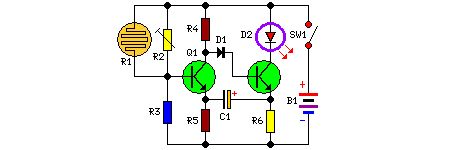# Learning Electronics

Learn to build electronic circuits

# Dark Activated Led or Lamp Flasher

Simple photo-sensitive circuit, 3V battery supply

This circuit adopts the rather unusual Bowes/White emitter coupled multivibrator circuit. The oscillation frequency is about 1Hz and is set by C1 value. The LED starts flashing when the photo resistor is scarcely illuminated. The onset of flashing can be set by trimming R2.

Circuit Diagram:Large View

Parts:

R1 = LDR
R2 = 100K
R3 = 10K
R5 = 470R
R6 = 47R
R4 = 10K
C1 = 220uF-25V
D1 = 1N4148
D2 = LED any type (see notes)
Q1 = BC337
Q2 = BC337
B1 = 3V Battery or 2x1.5V cells in series
SW1 = SPST Switch

Notes:
• Best results in flashing frequency can be obtained using for C1 a value in the 100 - 1000µF ranges.
• To drive a filament lamp make the following changes:
• Use a 2.2 to 3V, 250-300mA bulb in place of the LED
• R2 = 10K 1/2W Trimmer Cermet
• R3, R4 = 1K 1/4W Resistors
• R6 = 1R 1/4W Resistor
• C1 = 470 to 1000µF 25V Electrolytic Capacitor
• In LED-mode operation the stand-by current consumption is less than 400µA.
• In Lamp-mode operation the stand-by current consumption is about 3mA.

Source : www.redcircuits.com